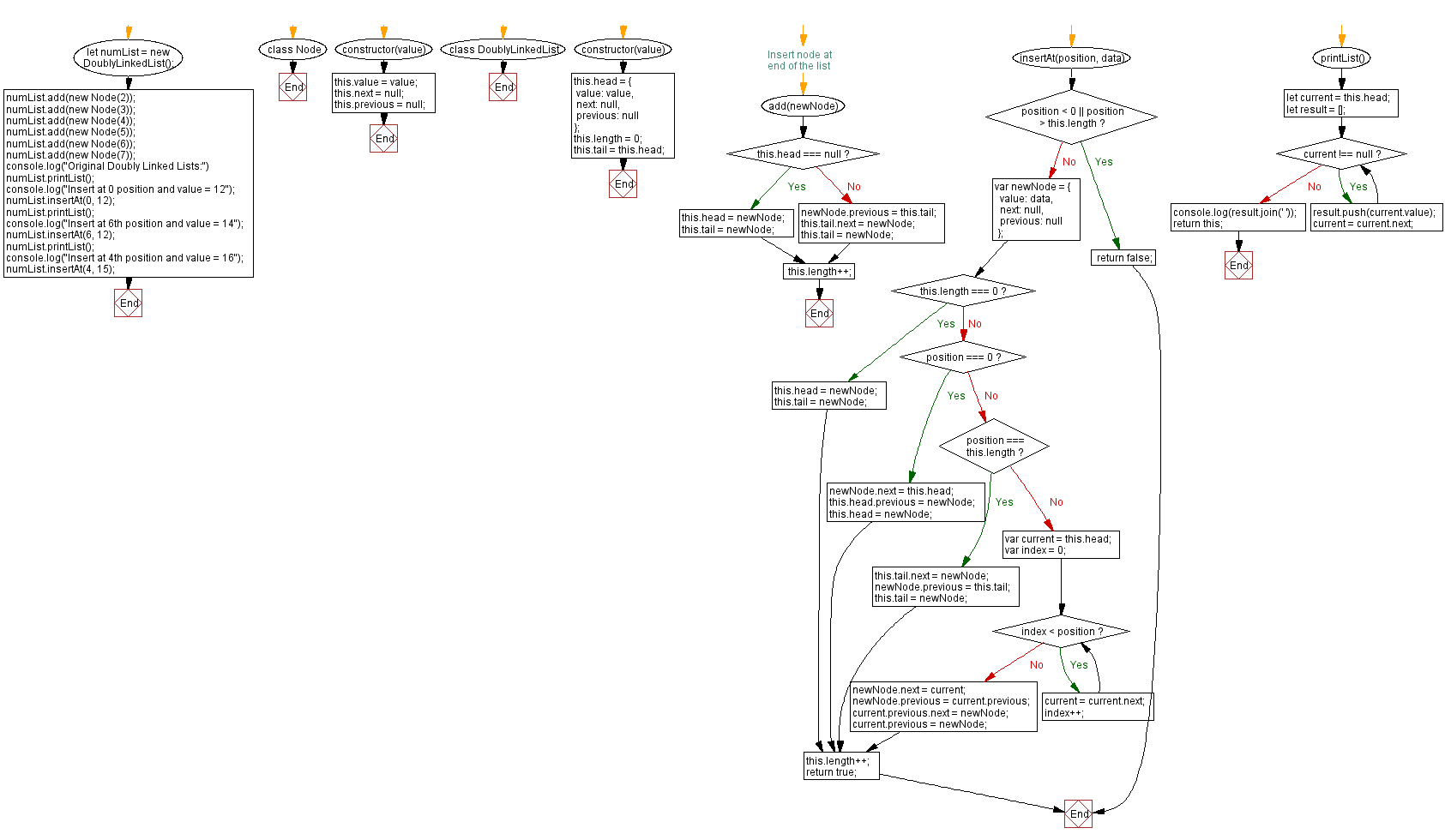# JavaScript Exercises: Insert a new node at any position of a Doubly Linked List

## JavaScript Data Structures: Exercise-5 with Solution

Write a JavaScript program to insert a new node at any position of a Doubly Linked List.

Sample Solution:

JavaScript Code:

``````class Node {
constructor(value) {
this.value = value;
this.next = null;
this.previous = null;
}
}

constructor(value) {
value: value,
next: null,
previous: null
};
this.length = 0;
}

// Insert node at end of the list

this.tail = newNode;
}
else
{
newNode.previous = this.tail;
this.tail.next = newNode;
this.tail = newNode;
}

this.length++;
}

insertAt(position, data) {
if (position < 0 || position > this.length) {
return false;
}

var newNode = {
value: data,
next: null,
previous: null
};

if (this.length === 0) {
this.tail = newNode;
} else if (position === 0) {
} else if (position === this.length) {
this.tail.next = newNode;
newNode.previous = this.tail;
this.tail = newNode;
} else {
var index = 0;
while (index < position) {
current = current.next;
index++;
}
newNode.next = current;
newNode.previous = current.previous;
current.previous.next = newNode;
current.previous = newNode;
}

this.length++;
return true;
}

printList() {
let result = [];
while (current !== null) {
result.push(current.value);
current = current.next;
}
console.log(result.join(' '));
return this;
}
}

numList.printList();
console.log("Insert at 0 position and value = 12");
numList.insertAt(0, 12);
numList.printList();
console.log("Insert at 6th position and value = 14");
numList.insertAt(6, 12);
numList.printList();
console.log("Insert at 4th position and value = 16");
numList.insertAt(4, 15);
```
```

Sample Output:

```Original Doubly Linked Lists:
2 3 4 5 6 7
Insert at 0 position and value = 12
12  2 3 4 5 6 7
Insert at 6th position and value = 14
12  2 3 4 5 12 6 7
Insert at 4th position and value = 16
```

Flowchart:Live Demo:

See the Pen javascript-doubly-linked-list-exercise-5 by w3resource (@w3resource) on CodePen.

Improve this sample solution and post your code through Disqus